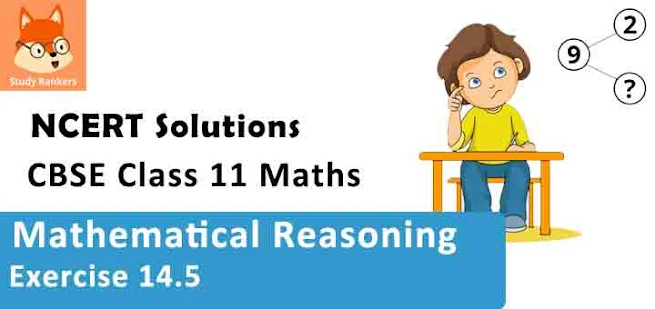# Class 11 Maths NCERT Solutions for Chapter 14 Mathematical Reasoning Exercise 14.5### Mathematical Reasoning Exercise 14.5 Solutions

1. Show that the statement
p: “If x is a real number such that x3 + 4x = 0, then x is 0” is true by
(i) direct method
(iii) method of contrapositive

Solution

p: “If x is a real number such that x3 + 4x = 0, then x is 0”.
Let q: x is a real number such that x3 + 4x = 0
r: x is 0.

(i) To show that statement p is true, we assume that q is true and then show that r is true.
Therefore, let statement q be true.
∴ x3 + 4x = 0
x (x2 + 4) = 0
⇒ x = 0 or x+ 4 = 0
However, since x is real, it is 0.
Thus, statement r is true.
Therefore, the given statement is true.

(ii) To show statement p to be true by contradiction, we assume that p is not true.
Let x be a real number such that x3 + 4x = 0 and let x is not 0.
Therefore, x3 + 4x = 0
x (x2 + 4) = 0
x = 0 or x2 + 4 = 0
x = 0 or x2 = – 4
However, x is real. Therefore, x = 0, which is a contradiction since we have assumed that x is not 0.
Thus, the given statement p is true.

(iii) To prove statement p to be true by contrapositive method, we assume that r is false and prove
that q must be false.
Here, r is false implies that it is required to consider the negation of statement r. This obtains the
following statement.
∼r: x is not 0.
It can be seen that (x2 + 4) will always be positive.
x ≠ 0 implies that the product of any positive real number with x is not zero.
Let us consider the product of x with (x2 + 4).
∴ x (x2 + 4) ≠ 0
⇒ x3 + 4x ≠ 0
This shows that statement q is not true.
Thus, it has been proved that
∼r ⇒ ∼q
Therefore, the given statement p is true.

2. Show that the statement “For any real numbers a and ba2 = b2 implies that a = b” is not true by giving a counter-example.

Solution

The given statement can be written in the form of “if-then” as follows.
If a and b are real numbers such that a2 = b2, then a = b.
Let p: a and b are real numbers such that a2 = b2.
q: a = b
The given statement has to be proved false. For this purpose, it has to be proved that if p, then ∼q.
To show this, two real numbers, a and b, with a2 = b2 are required such that a ≠ b.
Let a = 1 and b = –1
a2 = (1)2 = 1 and b2 = (– 1)2 = 1
∴ a2 = b2
However, a ≠ b
Thus, it can be concluded that the given statement is false.

3. Show that the following statement is true by the method of contrapositive.
p: If x is an integer and x2 is even, then x is also even.

Solution

p: If x is an integer and x2 is even, then x is also even.
Let qx is an integer and x2 is even.
rx is even.
To prove that p is true by contrapositive method, we assume that r is false, and prove that q is also false.
Let x is not even.
To prove that q is false, it has to be proved that x is not an integer or x2 is not even.
x is not even implies that x2 is also not even.
Therefore, statement q is false.
Thus, the given statement p is true.

4. By giving a counter example, show that the following statements are not true.
(i) p: If all the angles of a triangle are equal, then the triangle is an obtuse angled triangle.
(ii) q: The equation x2 – 1 = 0 does not have a root lying between 0 and 2.

Solution

(i) The given statement is of the form “if q then r”.
q: All the angles of a triangle are equal.
r: The triangle is an obtuse-angled triangle.
The given statement p has to be proved false. For this purpose, it has to be proved that if q, then ∼r.
To show this, angles of a triangle are required such that none of them is an obtuse angle.
It is known that the sum of all angles of a triangle is 180°. Therefore, if all the three angles are equal, then each of them is of measure 60°, which is not an obtuse angle.
In an equilateral triangle, the measure of all angles is equal. However, the triangle is not an obtuse-angled triangle.
Thus, it can be concluded that the given statement p is false.

(ii) The given statement is as follows.
q: The equation x2 – 1 = 0 does not have a root lying between 0 and 2.
This statement has to be proved false. To show this, a counter example is required.
Consider x2 – 1 = 0
x2 = 1
x = ± 1
One root of the equation x2 – 1 = 0, i.e. the root x = 1, lies between 0 and 2.
Thus, the given statement is false.

5. Which of the following statements are true and which are false? In each case give a valid reason for saying so.
(i) p: Each radius of a circle is a chord of the circle.
(ii) q: The centre of a circle bisects each chord of the circle.
(iii) r: Circle is a particular case of an ellipse.
(iv) s: If x and y are integers such that x > y, then –x < –y.
(v) t: √11 is a rational number.

Solution

(i) The given statement p is false.
According to the definition of chord, it should intersect the circle at two distinct points.

(ii) The given statement q is false.
If the chord is not the diameter of the circle, then the centre will not bisect that chord.
In other words, the centre of a circle only bisects the diameter, which is the chord of the circle.

(iii) The equation of an ellipse is,
x2 /a2 + y2 /b2  = 1
If we put a = b = 1, then we obtain
x2 + y2 = 1, which is an equation of a circle
Therefore, circle is a particular case of an ellipse.
Thus, statement r is true.

(iv) x > y
⇒ –x < –y (By a rule of inequality)
Thus, the given statement s is true.

(v) 11 is a prime number and we know that the square root of any prime number is an irrational number. Therefore, is an irrational number.
Thus, the given statement t is false.